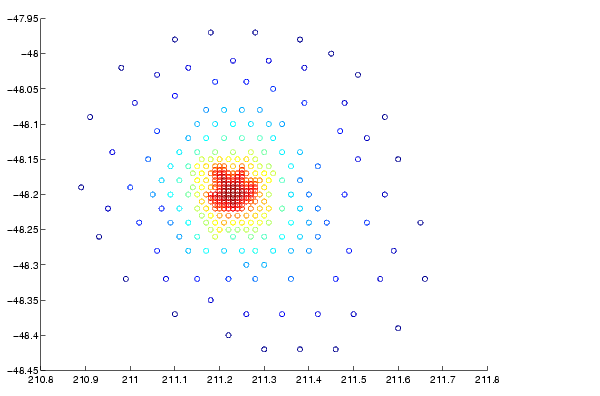MATLAB Function Referencescatter

2-D Scatter plot

Syntax

• ```scatter(X,Y,S,C)
scatter(X,Y)
scatter(X,Y,S)
scatter(...,markertype)
scatter(...,'filled')
h = scatter(...,)
```

Description

`scatter(X,Y,S,C)` displays colored circles at the locations specified by the vectors `X` and `Y` (which must be the same size).

`S` determines the area of each marker (specified in points`^2`). `S` can be a vector the same length as `X` and `Y` or a scalar. If `S` is a scalar, MATLAB draws all the markers the same size.

`C` determines the colors of each marker. When `C` is a vector the same length as `X` and `Y`, the values in `C` are linearly mapped to the colors in the current colormap. When `C` is a `length(X)`-by-3 matrix, it specifies the colors of the markers as RGB values. `C` can also be a color string (see `ColorSpec` for a list of color string specifiers)

`scatter(X,Y)` draws the markers in the default size and color.

`scatter(X,Y,S)` draws the markers at the specified sizes (`S`) with a single color.

`scatter(...,`markertype`)` uses the marker type specified instead of '`o`' (see `LineSpec` for a list of marker specifiers).

`scatter(...,'filled')` fills the markers.

`h = scatter(...)` returns the handles to the line objects created by `scatter` (see `line` for a list of properties you can specify using the object handles and `set`).

Remarks

Use `plot` for single color, single marker size scatter plots.

Examples

• ```load seamount
scatter(x,y,5,z)
````scatter3`, `plot`, `plotmatrix`save (serial) scatter3